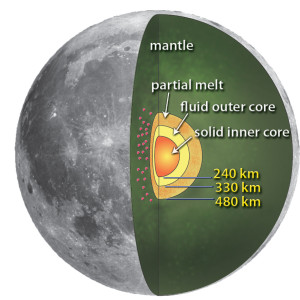# Energy of month redux

In the days since CNAV published an article on the change of the length of the month, something interesting happened. Walt Brown, originator of the Hydroplate Theory, realized he’d overlooked something. This often happens when one proposes a unifying theory to explain all the changes in our world, and the solar system, from one event. Especially an event as violent as the Global Flood.

Brown first wondered whether the moon had lost a fraction of its energy. Then he realized the moon need not have lost any energy at all. The month shortened in line with the decline of the mass of the earth. And that decline explains all the objects in the far reaches of our solar system.

# How the month and the earth’s mass relate

Recall the expression for the energy of the moon’s orbit:$E = - \dfrac{GMm}{2a}$

where M and m are the masses of the earth and the moon, G is Sir Isaac Newton’s gravitational constant, and a is the semimajor axis (the longest radius) of the orbit of the moon.

The month, or the period of the moon, relates to the semimajor axis thus:$P = 2 \pi \sqrt{\dfrac{a^3}{GM}}$

So that:$a = \sqrt{\dfrac{GMP^2}{4\pi^2}}$

So:$E = - m \sqrt{\dfrac{(\pi G)^2}{2}} \left ( \sqrt{M/P} \right )^2$

and thus:$E \alpha \left \lbrack \dfrac{M}{P} \right \rbrack ^ \frac{2}{3}$

## Loss of energy includes gain of heatCutaway of the moon showing the solid-fluid core, partial melt, and mantle, inferred from seismic data from Apollo 14, 15, 16, and 17. Diagram courtesy Renee Weber/NASA

In response to the last article, a regular commenter objected strongly to the notion that the moon lost 0.046 percent of its orbital energy. That would amount to about nine septillion joules. Impacts robbing the moon of that much energy might be only 50 percent efficient. So they would release at least nine septillion more joules, as heat. That could melt the moon, if not smash it to bits.

In fact, many meteoroids, large and small, bombarded the moon after the Flood. Most of these made the craters that all astronomers know. A few much larger ones made lava flow, to form the Great Basaltic Seas of the moon (Oceanus Procellarum and Maria Imbrium, Frigoris, Tranquillitatis, and Crisium on the near or “facing” side, Mare Humboltianum on the eastern edge, and Mare Moscoviense on the far side) and various “bays” of basalt. Obviously that bombardment did release heat, and a lot of it. In fact, the moon has solid and fluid cores, same as earth. But the modern observer has no reason to suppose all or even most of these rocks fell on one side (except for the impactors that formed the maria and sinuses).

# How sensitive is the month?

So, as Brown notes today, we have no reason to suppose the orbital energy of the moon changed at all. Therefore the ratio of the mass of the earth to the synodic month (new moon to new moon) did not change during the Flood. So:$\dfrac{M_{bf}}{M_{af}} = \dfrac{P_{bf}}{P_{af}}$

To express the pre-Flood month in pre-Flood days, one must convert by a factor of 360/365.256, the ratio of sidereal years in contemporary days before and after the Flood. So:$P_{bf} = \dfrac{M_{bf}}{M_{af}} P_{af} \times \left ( \dfrac{360}{365.256} \right )$

Substituting 1.03 for the mass ratio and 29.531 (modern) days for the modern synodic month gives an answer of 30 (ancient) days for the pre-Flood month. That’s actually precise to only two significant digits, because we do not (yet) know the mass ratio any more precisely than three significant digits.

# Where did all that mass go?Orbital elements of a satellite of the sun, like a TNO. Note the argument ω of perihelion, the largest bone of contention about the origins of TNOs. Courtesy of Walter T. Brown

The two to four percent of mass the earth lost, is the mass of the Trans-Neptunian Objects. How do we know that? Because in a key orbital element, the argument of perihelion, those objects are strikingly similar. (The argument of periapsis, abbreviated ω, is the arc an orbiting body passes through, after crossing a reference plane, to pass nearest the primary. “Periapsis” becomes “perihelion” when the primary is the sun.)

Megan E. Schwamb noted, in March of this year, that the twelve most distant TNOs all had the same argument of perihelion.

Any formation mechanism proposed for the origin of Sedna and 2012 VP113 [and the other ten most distant TNOs] will need to explain this orbital structure.

Dr. Robert B. Brown is a professor of astronautics at the United States Air Force Academy. (And, incidentally, one of Walter Brown’s sons.) He checked the orbital elements of all 1,346 TNOs. Their arguments of perihelion cluster near either 0° or 180°. The significance of that distribution, per the Chi-Square test, is 99.99 percent. Therefore, some large body, in or near the ecliptic plane, gave all those bodies their greatest push. (The ecliptic is the earth’s orbit around the sun. The plane of this orbit is the most common reference plane in the solar system.)

Which body was it? Not Neptune. Neptune would have been nowhere near most of these bodies in the last 22,000 years, if one assumed an old solar system. And this event must have happened recently. Otherwise all the TNO arguments of perihelion would have spread themselves out evenly. Which would, of course, be the null hypothesis, or what you would expect.

Only one other body remotely qualifies: the earth itself.

Total mass of the TNOs: from two to four percent of the earth’s mass, depending on whom you ask and how they count those objects.

This solves the riddle of how long the month was before the Global Flood, in relation to how long the month, and the day, are today.

## Related:

[subscribe2]

This site uses Akismet to reduce spam. Learn how your comment data is processed.